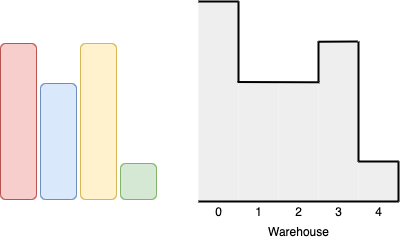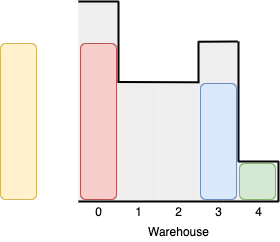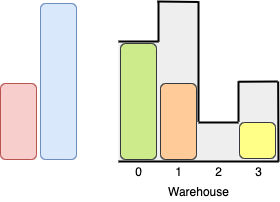# 1564. Put Boxes Into the Warehouse I

Given two arrays of positive integers `boxes` and `warehouse` representing the heights of some boxes of unit width, and the heights of `n` rooms in a warehouse, respectively. The warehouse's rooms are labeled from `0` to `n - 1` from left to right where `warehouse[i]` (0-indexed) is the height of the `ith` room.

Boxes are put into the warehouse by the following rules:

• Boxes can't be piled up.
• You can rearrange the order of the boxes.
• Boxes can only be pushed into the warehouse from left to right only.
• If the height of some room in the warehouse is less than the height of a box, then the box will be stopped before that room, so are the boxes behind it.

Return the maximum number of boxes you can put into the warehouse.

Example 1:```Input: boxes = [4,3,4,1], warehouse = [5,3,3,4,1]
Output: 3
Explanation:We can first put the box of height 1 in room 4. Then we can put the box of height 3 in either of the 3 rooms 1, 2, or 3. Lastly, we can put one box of height 4 in room 0.
There is no way we can fit all 4 boxes in the warehouse.```

Example 2:```Input: boxes = [1,2,2,3,4], warehouse = [3,4,1,2]
Output: 3
Explanation:Notice that it's not possible to put the box of height 4 into the warehouse since it cannot pass the first room of height 3.
Also, for the last two rooms, 2 and 3, only boxes of height 1 can fit.
We can fit 3 boxes maximum as shown above. The yellow box can also be put in room 2 instead.
Swapping the orange and green boxes is also valid, or swapping one of them with the red box.```

Example 3:

```Input: boxes = [1,2,3], warehouse = [1,2,3,4]
Output: 1
Explanation: Since the first room in the warehouse is of height 1, we can only put boxes of height 1.
```

Example 4:

```Input: boxes = [4,5,6], warehouse = [3,3,3,3,3]
Output: 0
```

Constraints:

• `n == warehouse.length`
• `1 <= boxes.length, warehouse.length <= 10^5`
• `1 <= boxes[i], warehouse[i] <= 10^9`

Medium

Prime

Unknown

### Solution(Chinese):

LEETCODE 1564. Put Boxes Into the Warehouse I 解题思路分析